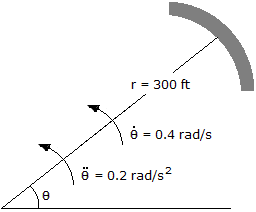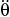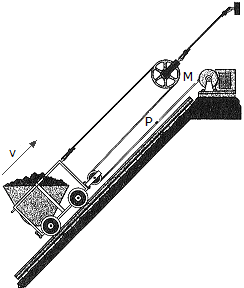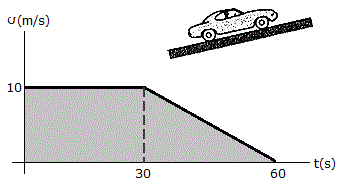# Engineering Mechanics - Kinematics of Particle (KOP)

### Exercise :: Kinematics of Particle (KOP) - General Questions

• Kinematics of Particle (KOP) - General Questions
21.A car is traveling along the circular curve of radius r = 300 ft. At the instant shown, its angular rate of rotation is= 0.4 rad / s, which is increasing at the rate of= 0.2 rad / s2. Determine the magnitude of the acceleration of the car at this instant.

 A. a = 108.0 ft/s2 B. a = 60.0 ft/s2 C. a = 48.0 ft/s2 D. a = 76.8 ft/s2

Explanation:

No answer description available for this question. Let us discuss.

22.The mine car is being pulled up to the inclined plane using the motor M and the rope-and-pulley arragement shown. Determine the speed vp at which a point P on the cable must be traveling toward the motor to move the the car up the plane with a constant speed of v = 5 m/s.

 A. VP = 1.667 m/s B. VP = 2.50 m/s C. VP = 15.00 m/s D. VP = 10.00 m/s

Explanation:

No answer description available for this question. Let us discuss.

23.A car travels up a hill with the speed shown in the graph. Compute the total distance the car moves until it stops at t = 60 s. What is the acceleration at t = 45 s?

 A. s = 400 m, a45 = -3.00 m/s2 B. s = 450 m, a45 = -0.333 m/s2 C. s = 400 m, a45 = -3.00 m/s2 D. s = 450 m, a45 = 0.333 m/s2

Explanation:

No answer description available for this question. Let us discuss.

24.A car is traveling along the circular curve of radius r = 300 ft. At the instant shown, its angular rate of rotation is= 0.4 rad / s, which is increasing at the rate of= 0.2 rad / s2. Determine the magnitude of the velocity of the car at this instant.

 A. v = 120.0 ft/s B. v = 0 C. v = 60.0 ft/s D. v = 169.7 ft/s

Explanation:

No answer description available for this question. Let us discuss.

25.

A particle is moving along a straight line through a fluid medium such that its speed is measured as v = (2t) m/s, where t is in seconds. If it is released from rest at s = 0, determine its positions and acceleration when t = 3 s.

 A. s = 9 m, a = 2 m/s2 B. s = 2 m, a = 18 m/s2 C. s = 18 m, a = 2 m/s2 D. s = 2 m, a = 9 m/s2